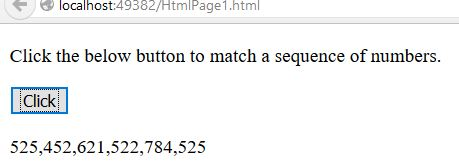# Matching sequence of numbers in JavaScript

##### How to match any string that contains a sequence of X (Digit) from specified digits in a string in JavaScript?

By using `d{X}` quantifier we can match any string that contains a sequence of X from specified digits in a string.

NOTE:

• \d is the Metacharacter, which is used to find a digit.
• {X} is the input value and it must be a number.
```<p>Click the below button to match a sequence of numbers.</p>
<input type="button" value="Click" onclick="mySearch()" />
<p id="myId"></p>

<script>
function mySearch() {
var a = "5252, 45286, 621522, 7845251";
var b = /\d{3}/g;
var r = a.match(b);
document.getElementById("myId").innerHTML = r;
}
</script>
```

In the above code snippet we have given `Id` as "`myId`"to the second `<p>` element in the HTML code. There is a function mySearch() in the`<script>`block which is connected to the onclick of the HTML button and there is a string with value "5252, 45286, 621522, 7845251" to the variable `a`, we need to match any string that contains a sequence of X from a specified digits, for that we are using `d{X}` quantifier in variable `b`.  `var b = /\d{3}/g` here `\d` is the metacharacter for finding a digit, 3 is the input character for matching a 3-digit sequence of numbers and `g` do the global search in a string, varaible `r` returns the matched sequence result. Onclick of the button "Click" in the HTML code fires the function mySearch() in the `<script>` block at the same time `d{X}` quantifier matches any string that contains a sequence of X (X=3) in a string with the help of global search `g` and gives the output.

OUTPUTViews: 2044 | Post Order: 146